Skip to main content Accessibility help
Home
Hostname: page-component-564cf476b6-r9chl Total loading time: 0.207 Render date: 2021-06-19T01:42:12.247Z Has data issue: true Feature Flags: { "shouldUseShareProductTool": true, "shouldUseHypothesis": true, "isUnsiloEnabled": true, "metricsAbstractViews": false, "figures": true, "newCiteModal": false, "newCitedByModal": true, "newEcommerce": true }Compositio Mathematica

# Strong orthogonality between the Möbius function, additive characters and Fourier coefficients of cusp forms

Published online by Cambridge University Press:  24 April 2014

Corresponding
E-mail address:

## Abstract

Let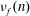$\nu _{f}(n)$ be the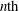$n\mathrm{th}$ normalized Fourier coefficient of a Hecke–Maass cusp form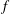$f$ for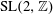${\rm SL }(2,\mathbb{Z})$ and let$\alpha$ be a real number. We prove strong oscillations of the argument of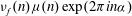$\nu _{f}(n)\mu (n) \exp (2\pi i n \alpha )$ as$n$ takes consecutive integral values.

## MSC classification

Type
Research Article
Information
Compositio Mathematica , May 2014 , pp. 763 - 797
Copyright
© The Author(s) 2014

## References

Bourgain, J., Sarnak, P. and Ziegler, T., Disjointness of Mobius from horocycle flows, in From Fourier analysis and number theory to radon transforms and geometry, Developments in Mathematics, vol. 28 (Springer, New York, 2013), 6783.CrossRefGoogle Scholar
Brumley, F., Maass cusp forms with quadratic integer coefficients, Int. Math. Res. Not. IMRN 18 (2003), 983997.CrossRefGoogle Scholar
Bump, D., Automorphic forms and representations, Cambridge Studies in Advanced Mathematics, vol. 55 (Cambridge University Press, Cambridge, 1998).Google Scholar
Carleson, L., On convergence and growth of partial sums of Fourier series, Acta Math. 116 (1966), 135157.CrossRefGoogle Scholar
Cellarosi, F. and Sinai, Y. G., Ergodic properties of square-free numbers, J. Eur. Math. Soc. (JEMS) 15 (2013), 13431374.CrossRefGoogle Scholar
Conrey, B. and Iwaniec, H., The cubic moment of central values of automorphic L-functions, Ann. of Math. (2) 151 (2000), 11751216.CrossRefGoogle Scholar
Davenport, H., On some infinite series involving arithmetical functions. II, Q. J. Math. 8 (1937), 313320.CrossRefGoogle Scholar
Duke, W., Friedlander, J. B. and Iwaniec, H., The subconvexity problem for Artin L-functions, Invent. Math. 149 (2002), 489577.CrossRefGoogle Scholar
Elliott, P. D. T. A., Multiplicative functions and Ramanujan’s τ-function, J. Aust. Math. Soc., Ser. A 30 (1980/81), 461468.CrossRefGoogle Scholar
Elliott, P. D. T. A., Moreno, C. J. and Shahidi, F., On the absolute value of Ramanujan’s τ-function, Math. Ann. 266 (1984), 507511.CrossRefGoogle Scholar
Gelbart, S. and Jacquet, H., A relation between automorphic representations of GL(2) and GL(3), Ann. Sci. Éc. Norm. Supér. (4) 11 (1978), 471542.CrossRefGoogle Scholar
Goldfeld, D., Automorphic forms and L-functions for the group GL(n, R), Cambridge Studies in Advanced Mathematics, vol. 99 (Cambridge University Press, Cambridge, 2006).Google Scholar
Goldfeld, D. and Li, X., Voronoi formulas on GL(n), Int. Math. Res. Not. IMRN (2006), 125; Art. ID. 86295.Google Scholar
Green, B. and Tao, T., The Möbius function is strongly orthogonal to nilsequences, Ann. of Math. (2) 175 (2012), 541566.CrossRefGoogle Scholar
Hoffstein, J. and Ramakrishnan, D., Siegel zeros and cusp forms, Int. Math. Res. Not. IMRN 6 (1995), 279308.CrossRefGoogle Scholar
Holowinsky, R., A sieve method for shifted convolution sums, Duke Math. J. 146 (2009), 401448.CrossRefGoogle Scholar
Iwaniec, H., Introduction to the spectral theory of automorphic forms, Rev. Mat. Iberoam. (1995).Google Scholar
Iwaniec, H., Topics in classical automorphic forms, Graduate Studies in Mathematics, vol. 17 (American Mathematical Society, Providence, RI, 1997).CrossRefGoogle Scholar
Iwaniec, H. and Kowalski, E., Analytic number theory, American Mathematical Society Colloquium Publications, vol. 53 (American Mathematical Society, Providence, RI, 2004).CrossRefGoogle Scholar
Kim, H., Functoriality for the exterior square of GL4and symmetric fourth of GL2. With Appendix 1 by D. Ramakrishnan, and Appendix 2 by H. Kim and P. Sarnak, J. Amer. Math. Soc. 16 (2003), 139183.CrossRefGoogle Scholar
Kim, H. and Shahidi, F., Functorial products for GL2×GL3and the symmetric cube for GL2. With an appendix by C.J. Bushnell and G. Henniart, Ann. of Math. (2) 155 (2002), 837893.CrossRefGoogle Scholar
Kim, H. and Shahidi, F., Cuspidality of symmetric power with applications, Duke Math. J. 112 (2002), 177197.Google Scholar
Lau, Y. K. and , G. S., Sums of Fourier coefficients of cusp forms, Q. J. Math. 62 (2011), 687716.CrossRefGoogle Scholar
Li, W., Newforms and functional equations, Math. Ann. 212 (1975), 285315.CrossRefGoogle Scholar
Liu, J., Wang, Y. and Ye, Y., A proof of Selberg’s orthogonality for automorphic L-functions, Manuscripta Math. 118 (2005), 135149.CrossRefGoogle Scholar
Liu, J. and Ye, Y., Selberg’s orthogonality conjecture for automorphic L-functions, Amer. J. Math. 127 (2005), 837849.CrossRefGoogle Scholar
Liu, J. and Ye, Y., Perron’s formula and the prime number theorem for automorphic L-functions, Pure Appl. Math. Q. 3 (2007), 481497; Special Issue: In honor of Leon Simon. Part 1.CrossRefGoogle Scholar
Miller, S., Cancellation in additively twisted sums on GL(n), Amer. J. Math. 128 (2006), 699729.CrossRefGoogle Scholar
Miller, S. D. and Schmid, W., Summation formulas, from Poisson and Voronoi to the present, in Noncommutative harmonic analysis, Progress in Mathematics, vol. 220 (Birkhäuser, Boston, MA, 2004), 419440.CrossRefGoogle Scholar
Miller, S. D. and Schmid, W., Automorphic distributions, L-functions, and Voronoi summation for GL(3), Ann. of Math. (2) 164 (2006), 423488.CrossRefGoogle Scholar
Montgomery, H. L. and Vaughan, R. C., Multiplicative number theory I. Classical theory, Cambridge Studies in Advanced Mathematics, vol. 97 (Cambridge University Press, Cambridge, 2007).Google Scholar
Murty, M. R., Oscillations of Fourier coefficients of modular forms, Math. Ann. 262 (1985), 431446.CrossRefGoogle Scholar
Murty, M. R. and Sankaranarayanan, A., Averages of exponential twists of the Liouville functions, Forum Math. 14 (2002), 273291.CrossRefGoogle Scholar
Perelli, A., On the prime number theorem for the coefficients of certain modular forms, in Elementary and analytic theory of numbers, Banach Center Publications, vol. 17 (PWN, Warsaw, 1982), 405410.Google Scholar
Rankin, R. A., Contributions to the theory of Ramanujan’s function τ (n) and similar arithmetical functions. I. The zeros of the function ∑n=1τ (n)∕n s on the line ℜs = 13∕2. II. The order of the Fourier coefficients of integral modular forms, Math. Proc. Cambridge Philos. Soc. 35 (1939), 357372.CrossRefGoogle Scholar
Rankin, R. A., An Ω result for coefficients of cusp forms, Math. Ann. 103 (1973), 239250.CrossRefGoogle Scholar
Rankin, R. A., Sum of powers of cusp form coefficients. II, Math. Ann. 272 (1985), 593600.CrossRefGoogle Scholar
Sarnak, P., Three lectures on the Möbius function randomness and dynamics. Available at www.math.ias.edu/files/wam/2011/PSMobius.pdf.Google Scholar
Sarnak, P. and Ubis, A., The horocycle flow at prime times. Preprint (2011),arXiv:1110.0777 [math.NT].Google Scholar
Selberg, A., Bemerkungen über eine Dirichletsche Reihe, die mit der Theorie der Modulformen nahe verbunden ist, Arch. Math. Naturvid. 43 (1940), 4750.Google Scholar
Soundararajan, K., Quantum unique ergodicity for SL2(ℤ)∖ℍ, Ann. of Math. (2) 172 (2010), 15291538.Google Scholar
Titchmarsh, E. C., The Theory of the Riemann zeta-function, second edition, (Clarendon Press, Oxford, 1986); revised by D. R. Heath-Brown.Google Scholar
Wu, J., Power sums of Hecke eigenvalues and application, Acta Arith. 137 (2009), 333344.CrossRefGoogle Scholar
Wu, J. and Xu, Z., Power sums of Hecke eigenvalues of Maass cusp forms, Ramanujan J., to appear.Google ScholarYou have Access
18
Cited by

# Send article to Kindle

To send this article to your Kindle, first ensure no-reply@cambridge.org is added to your Approved Personal Document E-mail List under your Personal Document Settings on the Manage Your Content and Devices page of your Amazon account. Then enter the ‘name’ part of your Kindle email address below. Find out more about sending to your Kindle. Find out more about sending to your Kindle.

Note you can select to send to either the @free.kindle.com or @kindle.com variations. ‘@free.kindle.com’ emails are free but can only be sent to your device when it is connected to wi-fi. ‘@kindle.com’ emails can be delivered even when you are not connected to wi-fi, but note that service fees apply.

Find out more about the Kindle Personal Document Service.

Strong orthogonality between the Möbius function, additive characters and Fourier coefficients of cusp forms
Available formats
×

# Send article to Dropbox

To send this article to your Dropbox account, please select one or more formats and confirm that you agree to abide by our usage policies. If this is the first time you use this feature, you will be asked to authorise Cambridge Core to connect with your <service> account. Find out more about sending content to Dropbox.

Strong orthogonality between the Möbius function, additive characters and Fourier coefficients of cusp forms
Available formats
×

# Send article to Google Drive

To send this article to your Google Drive account, please select one or more formats and confirm that you agree to abide by our usage policies. If this is the first time you use this feature, you will be asked to authorise Cambridge Core to connect with your <service> account. Find out more about sending content to Google Drive.

Strong orthogonality between the Möbius function, additive characters and Fourier coefficients of cusp forms
Available formats
×
×

#### Reply to:Submit a response

Please enter your response.

#### Your details

Please enter a valid email address.

#### Conflicting interests

Do you have any conflicting interests? *